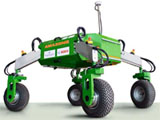## Biological Systems Engineering## Biological Systems Engineering: Papers and Publications

2001

#### Citation

Transactions of the ASAE, Vol. 44(3): 543–550

Copyright 2001 American Society of Agricultural Engineers

#### Abstract

Daily evapotranspiration (ET) rates are needed for irrigation scheduling. Owing to the difficulty of obtaining accurate field measurements, ET rates are commonly estimated from weather parameters. A few empirical or semi–empirical methods have been developed for assessing daily reference crop ET, which is converted to actual crop ET using crop coefficients. The FAO Penman–Monteith method, which is now accepted as the standard method for the computation of daily reference ET, is sophisticated. It requires several input parameters, some of which have no actual measurements but are estimated from measured weather parameters. In this study, we examined the suitability of fuzzy logic for estimating daily reference ET with simpler and fewer parameters. Two fuzzy evapotranspiration models, using two or three input parameters, were developed and applied to estimate grass ET. Independent weather parameters from sites representing arid and humid climates were used to test the models. The fuzzy estimated ET values were compared with direct ET measurements from grass–covered weighing lysimeters, and with ET estimations obtained using the FAO Penman–Monteith and the Hargreaves–Samani equations. The estimated ET values from a fuzzy model using three input parameters (Syx = 0.54 mm, r2 = 0.90) were found to be comparable to ET values estimated with the FAO Penman–Monteith equation (Syx = 0.50 mm, r2 = 0.91) and were more accurate than those obtained by the Hargreaves–Samani equation (Syx = 0.66 mm, r2 = 0.53). These results show that fuzzy evapotranspiration models with simpler and fewer input parameters can yield accurate estimation of ET.

COinS